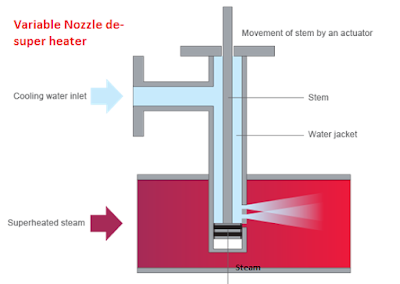### How to calculate ESP efficiency??

On what factors ESP efficiency depends on?

ESP efficiency depends on following factors.

• Dust collection area of ESP
• Dust particles size
• Dust or flue gas volume
• Resistivity of dust particles present in flue gas

What do you mean by Corona Power ratio?

Corona power ratio is the ratio of power consumed in watts to the flue gas flow in cubic feet per minute.

Corona power ratio = Power consumed by ESP in watts / Flue gas flow in CFM

This tells us about the energy consumed in filtering one cubic foot of flue gas per minute. The corona power ratio affects the efficiency of an electrostatic precipitator. Higher the corona power ratio, higher is the efficiency of the electrostatic precipitator

What do you mean by specific collecting area (SCA) & how do you calculate it?

It is the ratio of total collecting surface area of the ESP to the flue gas flow rate

SCA = Total collection area in m2 / Gas flow rate in m3/sec

SCA = m2/m3/sec

What do you mean by Aspect ratio in ESP?

It is the ratio of length of ESP to its Height

It should be in the range of 0.5 to 2

Calculation:

Calculate the aspect ratio of an ESP of total length 12 meter having its collecting plates height 11 meter.

Aspect ratio (AR) = Length of ESP / Height of ESP

Aspect ratio (AR) = 12/11 =1.09.

What is the treatment time in ESP having flue gas flow rate 90 m3/sec at velocity 0.7 m/sec.ESP having total 4 nos of fields each of length 3.5 meter.

We have, total length of ESP = 3.5 X 4 = 14 meter

Velocity of flue gas in ESP = 0.7 m/sec

So, total Treatment time for flue gas = 14 / 0.7 =20 seconds

Read power plant O&M reference books

An ESP consumes 7 KW power for treating flue gas of 17 m3/sec, calculate its Corona power ratio.

Corona power ratio = Power consumed by ESP in watts / Flue gas flow in CFM

We have flue gas flow = 17 m3/sec = 599.9 X 60= 35994 CFM

CPR = 7000 / 35994 = 0.2

An ESP handles total flue gas at the rate of 61920 m3 /h., its specific collecting surface area is 131.9 m2/m3/sec, calculate the efficiency of ESP if ash particles migration velocity is 5.46 cm/sec.

Solution:

Given that,

Flue gas flow in ESP, Q = 61920 m3/hr / 3600 = 17.2 m3/sec

Total collecting area A= 131.9 X 17.2 =2268.68 m2

Migration velocity of dust particles, V = 5.46/100 = 0.0546 m/sec

Efficiency of ESP = 1–eˆ (-AV/Q) X 100

= 1– eˆ (-2268.68 X 0.0546/17.2) X 100

ηESP = 99.92%

Read more>>> for such calculations powerplant & Calculations